telescopeѲptics.net          ▪▪▪▪                                             CONTENTS

# 10.2.4. Full-aperture Houghton corrector

PAGE HIGHLIGHTS
Houghton camera, symmetrical   • Calculating lens radii   • Example

Yet another type of a full-aperture lens corrector for spherical mirror uses an afocal pair of near-contact lenses. The lens surfaces are spherical. The idea surfaced at about the same time as for Maksutov-Bowers corrector, 1941 in Germany, published by Richter and Slevogt, and 1944 in the U.S. by Houghton. The Houghton corrector - as it is commonly referred to in the U.S. - is easier to fabricate than either Schmidt or meniscus-type corrector. For no obvious reason, it is apparently neglected in both, amateur and commercial telescopes.

Although this kind of corrector is not necessarily limited to only two lens elements, it is already capable of excellent correction in that form. Being afocal, the Houghton lens pair is theoretically free from secondary spectrum, but in practice it does have some residual longitudinal chromatism. It is negligible with moderately fast (slower than ~ƒ/3) systems, even when both elements are made of the same glass type. The chromatism can be significantly reduced by having lens element made of slightly different glass types (achromatized Houghton). With mirror's faster than ~ƒ/3, residual higher order spherical, as well as secondary spectrum, may become unacceptably large, although the former is significantly lower than in the Maksutov-type corrector.

Lens surfaces of the Houghton corrector are moderately curved, and the lens shape is quite flexible. The two easy to make forms of Houghton corrector - which makes them interesting in ATM circles - are a pair of either bi-convex/concave elements with two equal, reversed in sign curvatures - hereafter referred to as symmetrical (due to its reverse radii symmetry) type (FIG. 191a) - or a pair of plano-convex/concave elements, also with identical, reversed in sign surface curvatures, a plano-symmetrical type (FIG. 191b). The former offers advantage of both, spherical aberration and coma being corrected for any corrector position. The latter is easier to fabricate and has lower chromatism, but leaves residual coma - which may and may not be significant - unless placed at appropriate separation from the mirror, which may not be practical, or suitable for a particular system.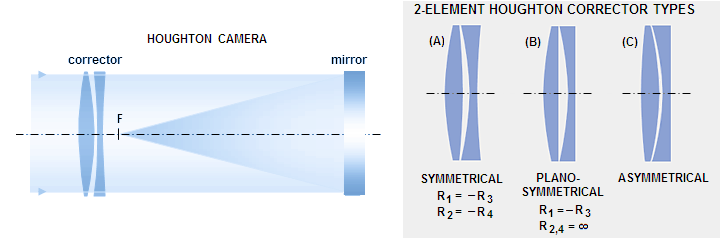FIGURE 191: RIGHT: Full-aperture Houghton corrector consists of a pair of lens elements generating spherical aberration and coma of the opposite sign to that of the mirror. This results in zero combined spherical aberration and coma. A zero-power corrector doesn't generate any significant level of astigmatism, so that mirror astigmatism can be influenced mainly by manipulating the aperture stop (i.e. corrector) location. Another advantage of a zero-power corrector is generally low secondary spectrum, although it may become significant (spherochromatism as well) with mirrors faster than ~ƒ/3. LEFT: The three basic lens configuration with Houghton corrector are: (A) with equal radii of reversed signs on the two lenses, hereafter called symmetrical, (B) same as the former, but with the 2nd and 4th surface flat, called plano-symmetrical, and (C) radii unrelated, hereafter asymmetrical Houghton corrector.

However, best overall correction in the 2-element version is obtainable with Houghton corrector having all four surface radii different (asymmetrical type FIG. 191c). This can become important as mirror relative aperture exceeds ~ƒ/3, with significant increase in secondary spectrum, spherochromatism and residual spherical aberration of the corrector. For slower mirrors, either symmetrical or plano-symmetrical Houghton corrector is all that is needed for excellent color correction.

While the Houghton corrector has originated as a single mirror (sphere) corrector - a Houghton camera - like its Schmidt and Maksutov counterparts, it can also be used in more complex system, the most interesting possibility being a two-mirror Houghton telescope.

10.2.7.1. Houghton camera: symmetrical corrector

In combination with spherical primary mirror, Houghton corrector needs to be over-corrected in order to cancel the system aberration. Aberration coefficient of corrector's primary spherical aberration at the best focus can be obtained from  the thin-lens expressions for spherical aberration (Eq. 48-50). For a single-glass corrector, with focal lengths of the front and rear element being equal, and of opposite sign (ƒ1=-ƒ2), and the position factor for the front and rear element for object at infinity being p1=-1 and p2=1, respectively, the corrector aberration coefficient for lower-order spherical aberration is: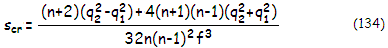with n being the refractive index, q1 and q2, as before, the front and rear lens shape factor (FIG. 192), respectively, and ƒ the single element focal length |ƒ| as an absolute (positive) value. Consequently, the P-V wavefront error at the best focus is, from Eq. 7, Wcr=scrD4/64, D being the aperture diameter. For the corrector type with lens curvatures equal but of opposite signs (either a pair of bi-convex/concave, or a pair of plano-convex/concave lenses), q1=q2=q, and the coefficient further simplifies to: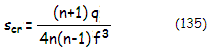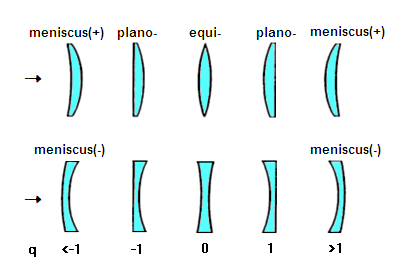FIGURE 192: Lens shape factor q=(R2+R1)/(R2-R1), with R1 and R2 being the front and rear lens radius of curvature, respectively, changes with the lens form and orientation in the standard Cartesian coordinate system (according to the sign convention, surface radius of curvature R is negative for the center of curvature to the left from the surface). While the choice of particular lens shape for a Houghton corrector is quite liberal, with no effect at the correction level of either mirror spherical aberration or coma, ease of fabrication favors either combination of a bi-convex and bi-concave lens of identical surface radii, or plano-convex with plano-concave lens arrangement. For ~ƒ/4 mirrors and slower, either gives excellent results. For ~ƒ/3 mirrors and faster, level of chromatism in the former, or coma in the latter, may make some other lens forms more suitable for the corrector (an option for significantly reducing chromatism is using two different glass types for the lens elements).

For zero 3rd order spherical aberration in combination with a mirror, the corrector aberration coefficient needs to be equal to that of the mirror, only of positive sign: scr=-(1+K)/4R3, where K is the mirror conic and R the mirror radius of curvature (negative). For spherical primary (K=0) and the corrector aberration coefficient scr given by Eq. 135, this fixes the corresponding shape factor q as: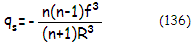R being the mirror radius of curvature. Any non-zero sum of the two coefficients ss = sm+scr will result in the system P-V error of spherical aberration at the best focus of Ws= ssD4/64. With q being positive, the corrector induces over-correction. Hence, if the corrector's contribution is stronger, the system will be over-corrected, and if weaker, the system will be under-corrected.

For given mirror radius of curvature, the choice of ƒ determines q, and the other way around. Choice of the shape factor q is normally determined by the zero coma aberration coefficient. The lower-order coma aberration coefficient for the corrector is, in general, given by: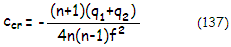and, for q1=q2=q (lens elements with equal curvatures of opposite sign), reduces to: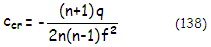with the P-V wavefront error of coma (from Eq.12 with θ set to 0) being Wcr= ccrαD3/12, α being the field angle in radians. For zero primary coma the corrector aberration coefficient needs to be nominally equal but of the opposite sign to the coma aberration coefficient of the mirror, given by cm= [1-σ(K1+1)]/R2, σ being the stop-to-mirror separation in units of the mirror radius of curvature, and K1 the primary conic. A non-zero system coefficient sum cs = cm+ccr will result in the system coma P-V wavefront error of Wc = csαD3/12.

For q1=q2=q, the zero coma shape factor q obtained from ccr=-cm is: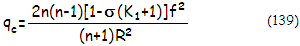which for K1=0 (spherical primary) simplifies to: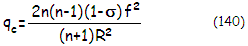Equating the shape factor qc needed for zero coma (Eq.140) with the shape factor qs needed for zero spherical aberration (Eq. 136) gives needed lens element focal length (positive or negative) in terms of the mirror radius of curvature R as:

|f|=2(1-σ)|R|              (141)

Eq. 141 shows that needed lens focal length for correction of lower-order spherical aberration and coma with the Houghton corrector becomes smaller as σ increases from 0 to 1, and Eq. 140 shows that the shape factor q also diminishes, with both focal length and q being nominally zero for σ=1. The reason is that as q diminishes to accommodate correction of coma decreasing with the increase in σ toward 1, relative difference in the radii for the two lenses also diminishes. The smaller radii difference, the more of a curvature (i.e. shorter focal length) needed to generate offset for the practically constant amount of spherical aberration of the mirror. For σ=1 there is no solution, since it requires zero corrector coma, which in turn requires a zero spherical-aberration equiconvex/equiconcave lens pair.

Substituting for ƒ in either expression for the shape factor q quantifies, with the refractive index n known, the specific lens shape factor needed for zero spherical aberration and coma. This quantity, in turn, determines needed lens radii ratio (absolute value) from: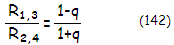Either radius expressed in terms of the other one and substituted in the lens focal length expression
ƒ=R
1R2/(n-1)(R2-R1), determines one of the radii, and the other one is then obtained from (Eq. 135). Taking, for instance, R1=(1-q)R2/(1+q) and substituting it into the focal length expression gives: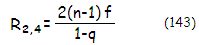with both lens radii of curvature Ri and lens focal length ƒ being absolute values.

This gives all the elements needed for an aplanatic Houghton system with symmetrical corrector type, either as a camera or in the Newtonian configuration with spherical primary (the later is sometimes incorrectly called Lurie-Houghton, which is an arrangement with aspheric primary), when the shape factor q is a free parameter. They can be summarized as follows:

 CALCULATING OPTICAL PARAMETERS FOR THE SYMMETRICAL HOUGHTON CORRECTOR (for mirror radius of curvature R) 1. Lens element focal length (absolute values for ƒ1,2 and ƒL): ƒ1,2 = 2(1-σ)|R| = ƒL σ=(corrector-to-mirror separation)/R 2. shape factor: q = n(n-1)(ƒL/R)3/(n+1)    = 8n(n-1)(1-σ)3/(n+1) 3. radius of curvature (absolute values for R1,2,3,4  and ƒL) 2nd and 4th surface: R2,4 = 2(n-1)ƒL/(1-q) 1st and 3rd surface: R1,3 = (1-q)R2/(1+q)        = 2(n-1)ƒL/(1+q) ▪ numerical signs for light traveling from left to right: R1,4>0 (positive), R2,3<0 (negative), R<0, ƒ1>0, ƒ2<0, and σ>0

Remaining lower-order aberrations are astigmatism and field curvature. Due to its zero-power, Houghton corrector induces near negligible astigmatism and field curvature. The dominant aberrations are those of the mirror (Eq. 18.3), modified by the stop position. Due to the aperture stop being moved from the primary to the corrector, system astigmatism is lower than that of the comparable paraboloid.

Astigmatism of a spherical mirror changes with (1-σ)2, where σ is, as before, corrector-to-mirror separation in units of mirror's radius of curvature. Change in astigmatism results in the change of median (best) field curvature, same as with the Schmidt-Newtonian (Eq. 111-112). Particular stop position will produce flat best image surface, with relatively low residual mirror astigmatism. For mirror alone, it occurs for σ=(1-0.5-K/2)/(1+K); assuming spherical mirror and near zero Houghton corrector astigmatism, the flat-field corrector (stop) position is closely approximated by σ=1-0.5=0.29. Since mirror astigmatism changes in proportion to (1-σ)2, the remaining astigmatism is about half of that for a mirror with the stop at the surface. However, σ~0.3 (i.e. 0.6 of the mirror focal length) would require large diagonal, so most of he actual instruments will have some residual, relatively mild field curvature, with astigmatism about a third that of the mirror.

EXAMPLE: Symmetrical Houghton corrector for 200mm ƒ/4 sphere, thus R=-1600mm. The absolute value for the focal length of the front (positive) and rear (negative) corrector lens is ƒ1,2 = -2(1-σ)R. Placing the corrector at 640mm from the mirror gives σ=0.4, ƒ1=1920mm and ƒ2=-1920mm. With n=1.5 for the e-line (K11 glass), the shape factor q=0.5184. The absolute value for the second and fourth corrector radii is R2,4=3987, with R2 positive and R4 negative. The absolute value for the first and third radius is R1,3=1264, with R1 positive and R3 negative.

Best image field curvature rc=R/[2 - 4(1-σ)2]=-2857mm.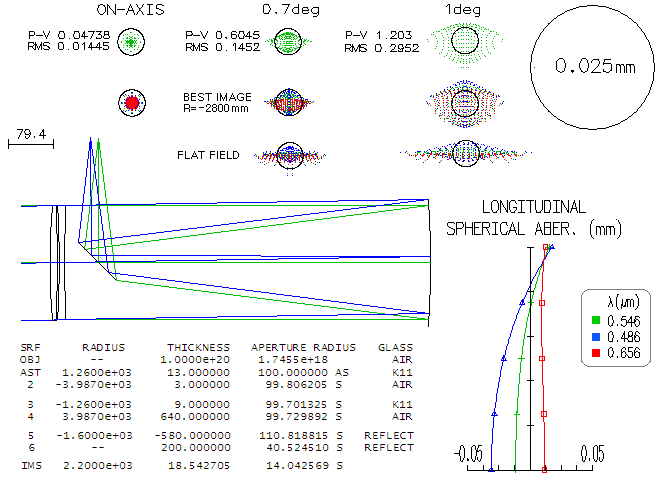Residual spherical aberration is less than 1/20 wave P-V. It can be removed by a slight weakening of the 1/3 radii, to +-1270mm, but it wouldn't make perceptible difference. Similarly, the blue and red can be optimally pulled closer together by a slight strengthening of 3rd radius (to 3925mm), without inducing spherical aberration, but it wouldn't be worth the effort at this error magnitude. As it is, the wavefront error is 0.18 and 0.12 wave P-V in the blue and red, respectively. There is no lateral color error to speak of.

Lens thickness should be at its reasonable minimum, for the minimum secondary spectrum, but this factor is not significant.

It is important to note that the corrector is very sensitive to tilt, either to both lenses tilted equally, or the rear lens alone. As little as 0.1° of tilt (1/3mm from top to bottom) would induce near 0.8 and 0.9 wave P-V of centerfield coma for both and only rear lens tilted, respectively. The front lens alone is much less sensitive: the same amount of tilt would induce less than 0.14 wave P-V of coma.